# Logic Operation AND

## AND Gate

In its most basic configuration, the gate has two inputs and one output. The output is one only when both inputs are one.
En su configuración más básica, la compuerta tiene dos entras y una salida. La salida es uno solamente cuando ambas entradas son uno.

 x1 x2 z 0 0 0 0 1 0 1 0 0 1 1 1

 Problem 1 Open Neural Lab and create a New Project called MappingAnd as shown. Abra Neural Lab y cree un Nuevo Proyecto llamado MappingAnd como se muestra.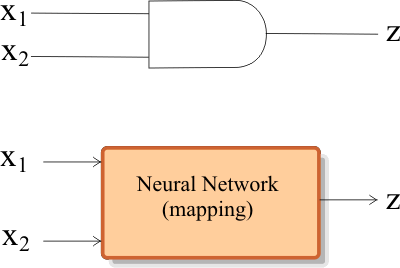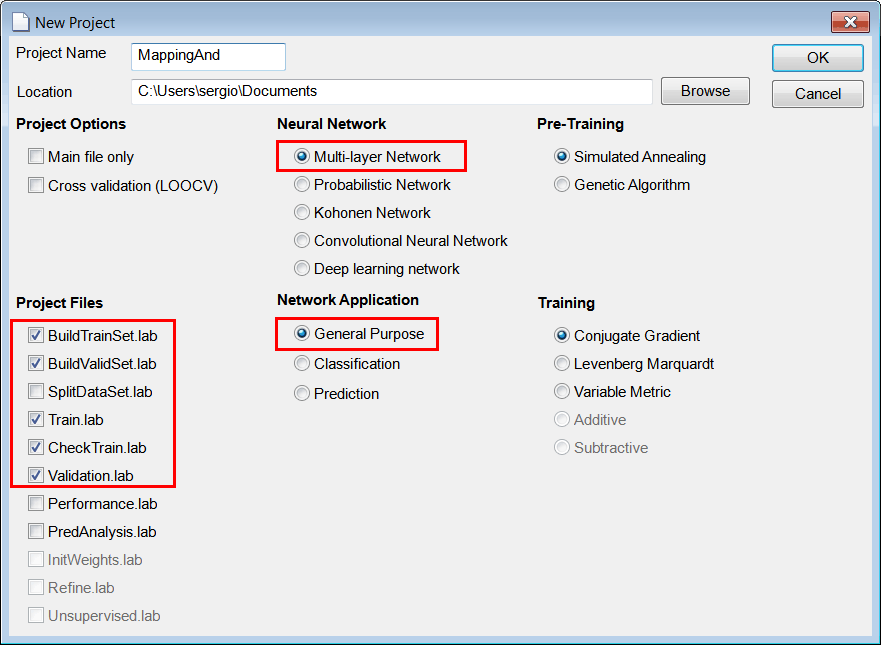Solution 1 Edit the BuildTrainSet.lab file to build an appropriate training set for the AND gate. Use 64 training cases.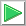Run click the button to execute the code. If you do not have any errors, the training set will be generated and displayed on the variable list and the file list. Click on the file to see its contents.

 MappingAnd\BuildTrainSet.lab int numCases = 64; Matrix input; input.CreateRandom(numCases, 2, 0.0, 2.0); // Random values in [0 2) input= floor(input);// Round any value in [0 1) to 0 and any value in [1 2) to 1 Matrix trainSetTarget; trainSetTarget.Create(numCases, 1); //_________________________________________ Compute the target for each case int row = 0; for(row =0; row < numCases; row++) {      if (input[row] == 1 && input[row] == 1)      {           trainSetTarget[row] = 1;      }      else      {           trainSetTarget[row] = 0;      } } //_________________________________________ Create some noise Matrix noise; noise.CreateRandom(numCases, 2, 0.0, 1.0); // Random values in [0 1) //_________________________________________ Contaminate the input with noise Matrix trainSetInput = 0.9 * input+ 0.1 * noise; //_________________________________________ Save the training set trainSetInput.Save(); trainSetTarget.Save();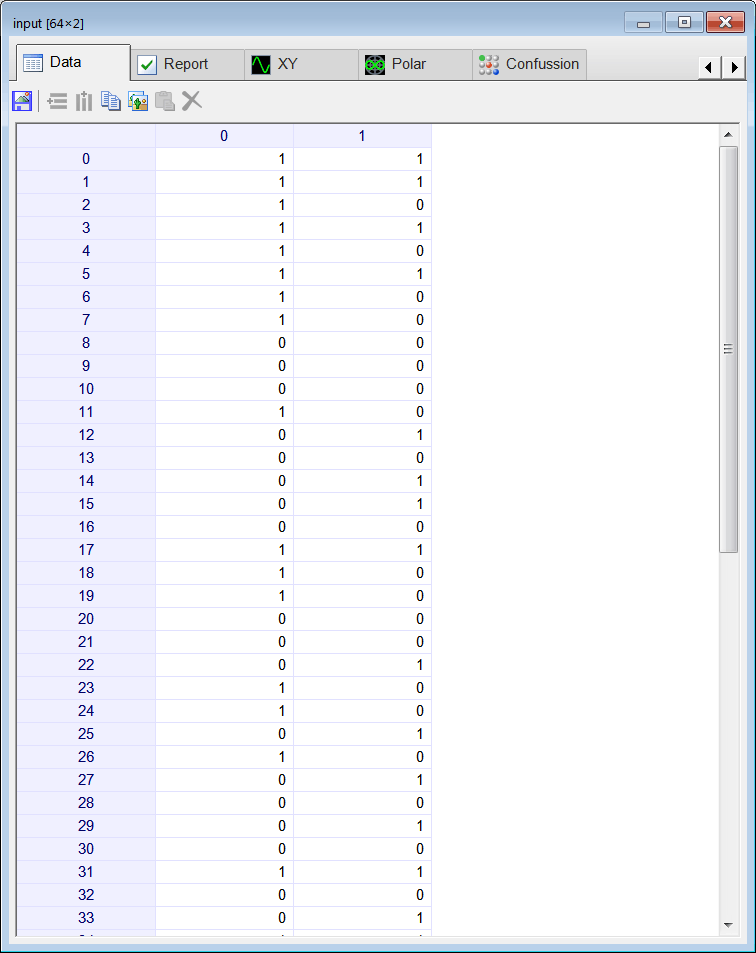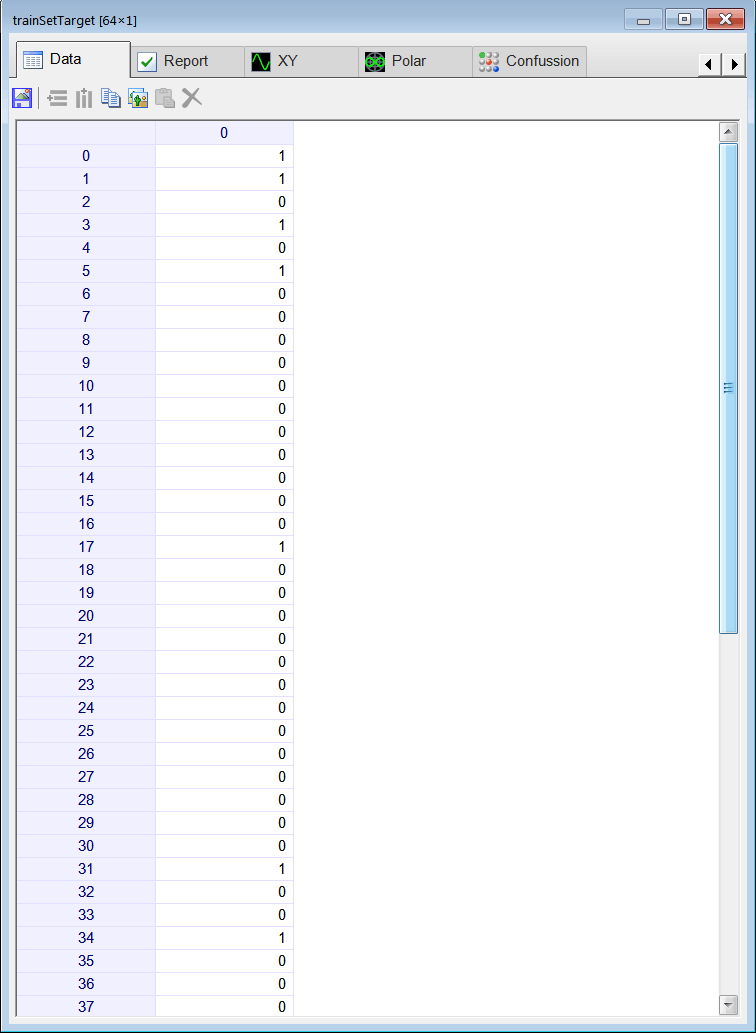Tip Observe that the values in the training set input are random values between zero and one without including values from 0.1 to 0.9.Observe que los valores en el conjunto de entrenamiento de entrada son valores aleatorios entre cero y uno sin incluir los valores desde 0.1 a 0.9.

 Problem 2 Open the BuildValidSet.lab file to create a validation set for the AND gate. Use 128 validation cases.

 Solution 2 Edit the file as shown, thenRun click the button to execute the code. If you do not have any errors, the validation set will be generated and displayed on the variable list and the file list. Click on the file to see its contents. Note that in this specific case the training set and the validation set were built similarly.

 MappingAnd\BuildValidSet.lab int numCases = 128; Matrix input; input.CreateRandom(numCases, 2, 0.0, 2.0); // Random values in [0 2) input= floor(input); // Round any value in [0 1) to 0 and any value in [1 2) to 1 Matrix validSetTarget; validSetTarget.Create(numCases, 1); //_________________________________________ Compute the target for each case int row = 0; for(row =0; row < numCases; row++) {      if (input[row] == 1 && input[row] == 1)      {           validSetTarget[row] = 1;      }      else      {           validSetTarget[row] = 0;      } } //_________________________________________ Create some noise Matrix noise; noise.CreateRandom(numCases, 2, 0.0, 1.0); // Random values in [0 1) //_________________________________________ Contaminate the input with noise Matrix validSetInput = 0.9 * input+ 0.1 * noise; //_________________________________________ Save the validation set validSetInput .Save(); validSetTarget.Save();

 Problem 3 Indicate whether the following statement is true or false: The target must also be contaminated with noise for best performance.

 Problem 4 Design and train an ANN for the AND gate. Use one hidden layer and one neuron in this layer. Train the ANN using simulated annealing as shown:      Number of iterations = 100      Number of Temperatures = 100      Initial Temperature = 15      Final Temperature = 0.01      Cooling Schedule = Linear      Number of Cycles = 4      Goal (desired mse) = 0.00001 Neural Lab includes other training methods. Any training method or any combination of them may be used to train an ANN. Some methods are faster than others; and some methods require more memory than others. Please note that simulated annealing is an optimization method inspired on the process of annealing that in this specific case is used for ANN training.

 Solution 4 Open the Train.lab file and edit the file as shown. Then,Run click the button to execute the code. If you do not have any errors, the ANN will be trained when the code execution stops. Double click the network in the variable list to see the network. At the top of the network dialog, select the inputTrainSet.csv file and the trainSetTarget.csv file as shown. You will be able to see the network behavior in real time by moving the scrollbar at the right.

 MappingAnd\Train.lab //_________________________________________ Network Setup LayerNet net; net.Create(2, 1, 0, 1); net.SetInScaler(0, 0.0, 1.0); // Input 0: values are from 0.0 to 1.0 net.SetInScaler(1, 0.0, 1.0); // Input 1: values are from 0.0 to 1.0 net.SetOutScaler(0, 0.0, 1.0); // Output 0: values are from 0.0 to 1.0 //_________________________________________ Load and set the training set Matrix trainSetInput; trainSetInput.Load(); Matrix trainSetTarget; trainSetTarget.Load(); net.SetTrainSet(trainSetInput, trainSetTarget, false); //_________________________________________ Train using Simulated Annealing //net.TrainSimAnneal( //     100, //Number of Temperatures //     100, //Number Iterations //     15, //Initial Temperature //     0.01, //Final Temperature //     true, // Is Cooling Schedule Linear? //     4, // Number of Cycles //     1.0e-5 // Goal (desired mse) //); //________________________ Train using Genetic Algorithms net.TrainGenetic(      200, // Population Size      200, //Number Generations      1.5, // Over Population      0.001, // Probability of Mutation      0.8, // Probability of Crossover      1.0e-12); // Goal (desired mse) //_________________________________________ Save the trained network net.Save();

 Hint The methods SetInScaler and SetOutScaler of the network take three parameters. The first indicates the input or output index respectively. The second indicates the minimum value. The third indicates the maximum value. Los métodos de la red SetInScaler y SetOutScaler toman tres parámetros. El primero indica el índice de la entrada o de la salida respectivamente. El segundo indica el valor mínimo. El tercero indica el valor máximo.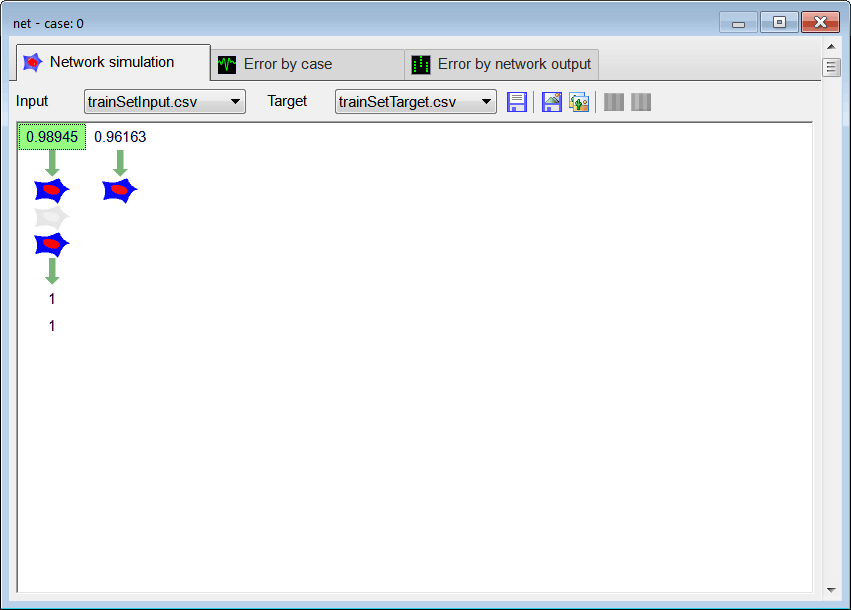Tip In the code shown the ANN was trained using simulated annealing. However, other training methods can be used. Each training method has its advantages and disadvantages. The training parameters used are optimization values that will be discussed later.

 Problem 5 Edit the CheckTraining.lab file to check the training: (a) Compute the mean squared error for the ANN using the training set. (b) Plot the error for each training case. (c) Save the plot as a vector image (checkTraining.pdf and checkTraining.emf)

 Solution 5 (a) After editing the file,Run click the button to execute the code. If you do not have any errors, the mse will be displayed in the variable list.

 MappingAnd\CheckTraining.lab //_________________________________________ Load the training set Matrix trainSetInput; trainSetInput.Load(); Matrix trainSetTarget; trainSetTarget.Load(); //_________________________________________ Load the ANN LayerNet net; net.Load(); //_________________________________________ Run Matrix output = net.Run(trainSetInput); double mse = ComputeMse(output, trainSetTarget); //_________________________________________ Relative Error Vector error = ComputeRelError(output, trainSetTarget); XyChart checkTraining; checkTraining.SetCaption("case", false, "Relative Error", false); checkTraining.AddGraphY(error, "Relative Error", 2, 1, 0, 255, 0); checkTraining.SetLogScale(false, true); checkTraining.SetLimits(0, trainSetTarget.GetRowCount(), 1.0e-10, 1.0); checkTraining.SetColorMode(2); checkTraining.SaveAndShow(); checkTraining.SavePDF(0.0); checkTraining.SaveEMF();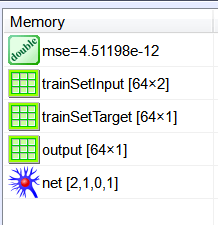Solution 5 (b) Double click the network in the variable list to see the network. Then select the trainSetInput.csv file and the trainSetTarget.csv file.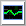Error by case click the tab to plot the error for each training case.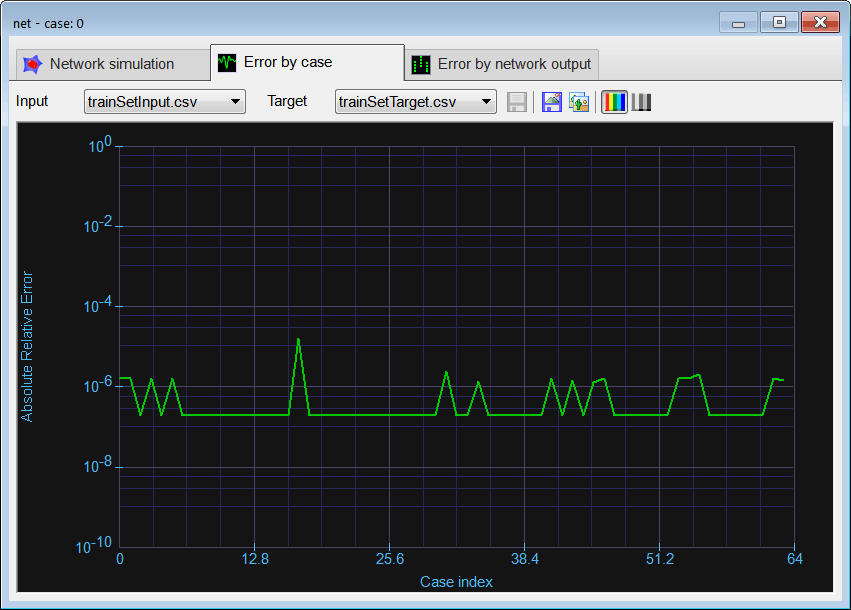Problem 6 Open the Validation.lab file to perform the validation of the ANN. (a) Compute the mean squared error for the ANN using the validation set. (b) Plot the error for each validation case. (c) Save the plot as a vector image (validation.pdf and validation.emf)

 Solution 6 (a) After editing the Validation file,Run click the button to execute the code.. If you do not have any errors, the mse will be displayed in the variable list. As the value of the mse for both the training set and the validation set is similar, the ANN has been properly trained.

 Validation.lab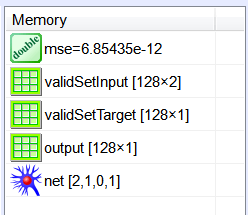Solution 6 (b) Double click the network in the variable list to see the network, select the validSetINput.csv file and the validSetTarget.csv file.Error by case click the button to plot the error for each training case.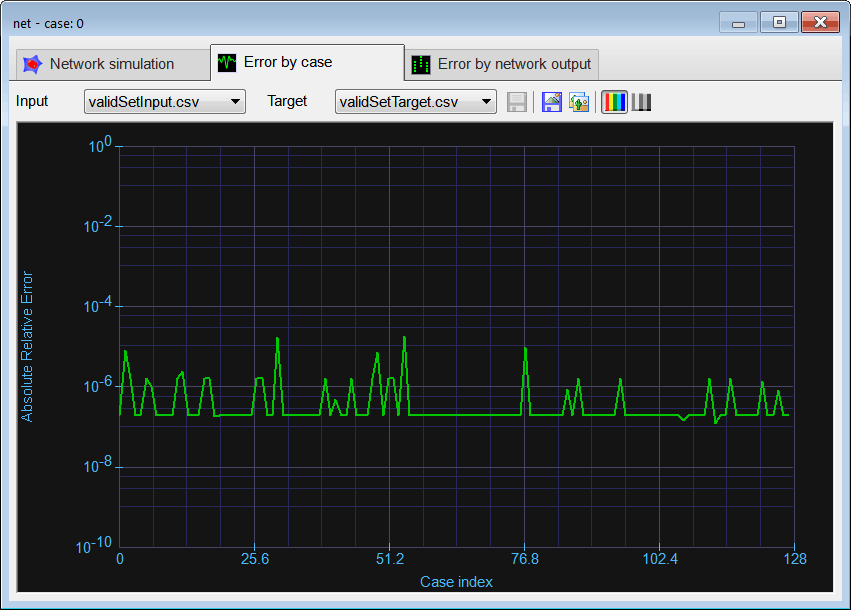Tip Most ANN problems can be organized in five steps implying five files. The figure below shows the name of each code and the files generated when the code is executed.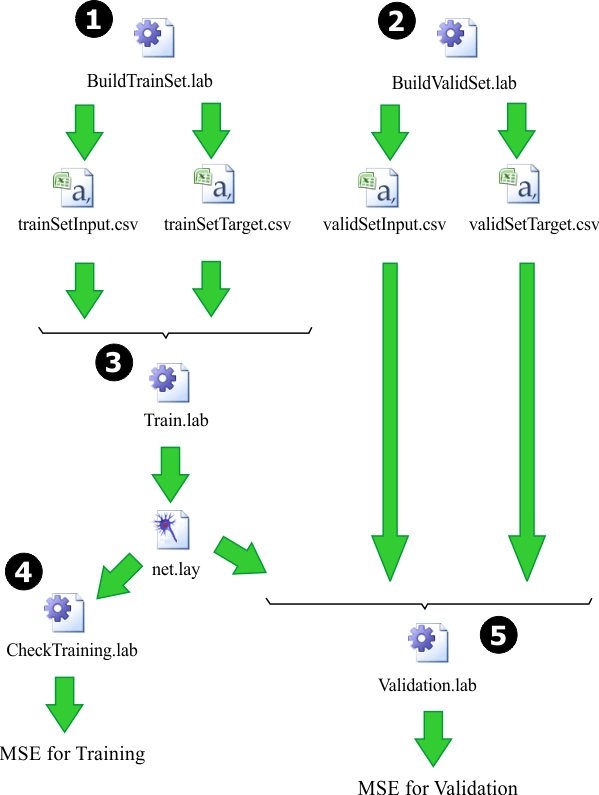Problem 7 Generate a report in Microsoft Word. Write some conclusions in the report focusing on the problems that were faced during the simulation and how these problems were or could be solved. Observe that you can insert EMF files into a Microsoft Word Document. Observe also that you cannot insert PDF files into a Microsoft Word Document.

 Solution 7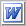Create Report click the button to generate a report in Microsoft Word. Set the name to MyReport.docx and save the report. You may edit the file to suit your requirements.

 Tip The final product of these problems is a trained neural network that can be used in a real application to solve a specific problem.